1. Capacitance of a capacitor is defined as the
2. A battery is used to charge a capacitor fully through a resistor of resistance R. The energy supplied by the battery is

Eb. The energy stored by the capacitor is Ec.

What is the relationship between Eb and Ec?

3. An alternating current is sinusoidal and has a maximum value of 1.5 A. What is the approximate value of the root mean squared (rms) current?
4. A magnetic field of strength B links a coil. The direction of the field is normal to the plane of the coil. The graph shows how B varies with time t.Which of the following graphs shows how the induced emfε in the coil varies with t?

5. Which of the following experiments provides evidence for the existence of matter waves?
6. Three identical capacitors, each of capacitance C, are connected as shown.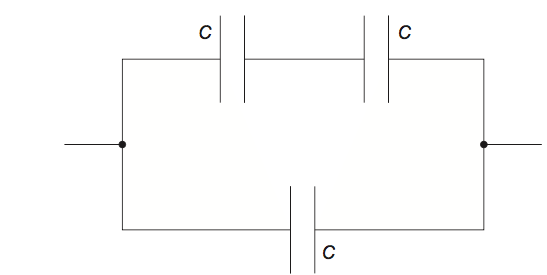What is the total capacitance of the combination?

7. A capacitor is charged by a constant current of 2.5 μA for 100 s. As a result the potential difference across the capacitor increases by 5.0 V. What is the capacitance of the capacitor?
8. An alternating current generator produces a root mean squared (rms) emf of ε at a frequency f. The rotational speed of the coil in the generator is doubled. Which of the following correctly identifies the new output rms emf and the new frequency?9. The voltage output of a particular power station is stepped up by a factor of 103. As a result the power loss in the transmission cables is reduced by a factor of
10. An ideal transformer has a primary coil with

Np turns and a secondary coil with Ns turns. The electrical power input to the primary is P. Which of the following is the power output from the secondary?

11. An aircraft with a wing span of 50 m flies horizontally at a speed of 200

ms−1. The vertical component of the Earth’s magnetic field at the plane’s position is 10 μT.What electromotive force (emf) is induced between points A and B on the aircraft?

12. Three capacitors, each one with a capacitance C, are connected such that their combined capacitance is 1.5C. How are they connected? M18/4/PHYSI/HPM/ENG/TZ2/36
13. The graph shows the power dissipated in a resistor of 100 Ω when connected to an alternating current (ac) power supply of root mean square voltage

(Vrms) 60 V.What are the frequency of the ac power supply and the average power dissipated in the resistor?14. Raoul suggests that power losses in a transformer may be reduced by the following.

I.     Constructing the core from a solid block of steel.

II.     Using large diameter wire in the coils.

III.     Using wire of low resistivity.

Which of the above suggestions would reduce power loss?

15. A rectangular flat coil moves at constant speed through a uniform magnetic field. The direction of the field is into the plane of the paper.Which graph shows the variation with time t, of the induced emf ε in the coil as it moves from P to Q?

16. A copper sheet is suspended in a region of uniform magnetic field by an insulating wire connected to a horizontal support. The sheet is pulled to one side so that it is outside the region of the field, and then released.The uniform magnetic field is directed into the plane of the paper.

Which of the following is true for both the direction of the induced current in the sheet and the change in amplitude of the oscillations of the sheet with time?17. Three conducting loops, X, Y and Z, are moving with the same speed from a region of zero magnetic field to a region of uniform non-zero magnetic field.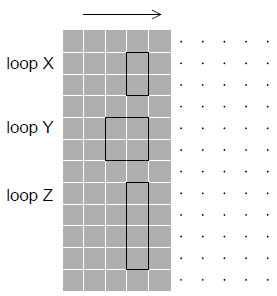Which loop(s) has/have the largest induced electromotive force (emf) at the instant when the loops enter the magnetic field?

18. An alternating current (ac) generator produces a peak emf

E0 and periodic time T. What are the peak emf and periodic time when the frequency of rotation is doubled?19. Six identical capacitors, each of value C, are connected as shown.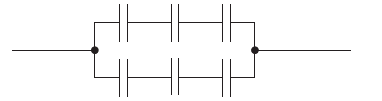What is the total capacitance?

20. The current I flowing in loop A in a clockwise direction is increasing so as to induce a current both in loops B and C. All three loops are on the same plane.What is the direction of the induced currents in loop B and loop C?21. The ratiofor a transformer is 2.5.

The primary coil of the transformer draws a current of 0.25 A from a 200 V alternating current (ac) supply. The current in the secondary coil is 0.5 A. What is the efficiency of the transformer?

22. The rms voltage of a sinusoidal electricity supply is 110V. The maximum potential difference during one cycle is
23. A rectangular loop of conducting wire rotates in a region of magnetic field. The graph shows the variation with time t of the induced emf in the loop during one cycle.The resistance of the coil is 5.0 Ω

. Which of the following is the average power dissipated in the loop?

24. A flat coil with N turns has a cross-sectional area A. The coil has a flux density of B in a direction of 90º to the plane of the coil.

What is the magnetic flux linkage?

25. The rms current rating of an electric heater is 4A. What direct current would produce the same power dissipation in the electric heater?
26. In order to reduce power losses in the transmission lines between a power station and a factory, two transformers are used. One is located at the power station and the other at the factory. Which of the following gives the correct types of transformer used?27. A permanent bar magnet is moved towards a coil of conducting wire wrapped around a non-conducting cylinder. The ends of the coil, P and Q are joined by a straight piece of wire.The induced current in the straight piece of wire is

28. Faraday’s law of electromagnetic induction states that the electromotive force (emf) induced in a conductor is proportional to
29. The plane of a coil is positioned at right angles to a magnetic field of flux density B. The coil has N turns, each of area A. The coil is rotated through 180˚ in time t.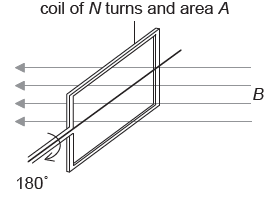What is the magnitude of the induced emf?

30. A parallel-plate capacitor is connected to a cell of constant emf. The capacitor plates are then moved further apart without disconnecting the cell. What are the changes in the magnitude of the electric field between the plates and in the capacitance of the capacitor?31. Three capacitors are arranged as shown.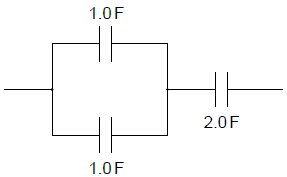What is the total capacitance of the arrangement?

32. A direct current (dc) of 5A dissipates a power P in a resistor. Which peak value of the alternating current (ac) will dissipate an average power P in the same resistor?
33. In an ideal transformer

I. the power output exceeds the power input
II. the magnetic flux produced by the primary coil entirely links the secondary coil
III. there are more turns on the secondary coil than on the primary coil.

Which of the above statements must be true?

34. A rectangular loop of conducting wire rotates in a region of magnetic field. The graph shows the variation with time t of the induced emf in the loop during one cycle.Which of the following gives the correct times at which the magnitude of the magnetic flux linkage and the magnitude of the current in the loop are maximum?35. A length of copper wire PQ is moved downwards through the poles of two horizontal bar magnets as shown below.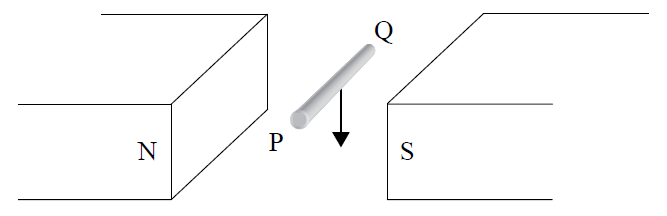Compared to end Q, end P will have

36. The diagram shows a conducting rod of length L being moved in a region of uniform magnetic field B. The field is directed at right angles to the plane of the paper. The rod slides on conducting rails at a constant speed v. A resistor of resistance R connects the rails.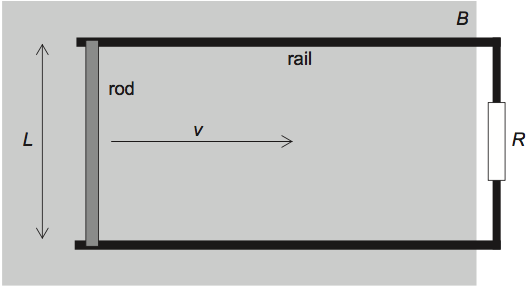What is the power required to move the rod?

37. The capacitance of a device is defined as the
38. The capacitance of a pixel of a CCD is 3.2 pF. A pulse of light is incident on the pixel and as a result,

104 electrons are ejected from the pixel. The magnitude of the change in potential of the pixel is

39. What are the units of magnetic flux and magnetic field strength?40. Which of the following reduces the energy losses in a transformer?
41. Two identical resistors R are connected in series to an alternating current (ac) power supply. The power supply has a root mean squared (rms) voltage of V and an rms current of I. What is the maximum power developed in one of the resistors in the circuit?
42. An alternating current supply of negligible internal resistance is connected to two resistors that are in parallel.The resistance of each resistor is R and the peak voltage of the ac supply is V0. Which of the following is the average power dissipated in the circuit?

43. The graph shows the variation with time t of the magnetic flux φ through a coil that is rotating in a uniform magnetic field.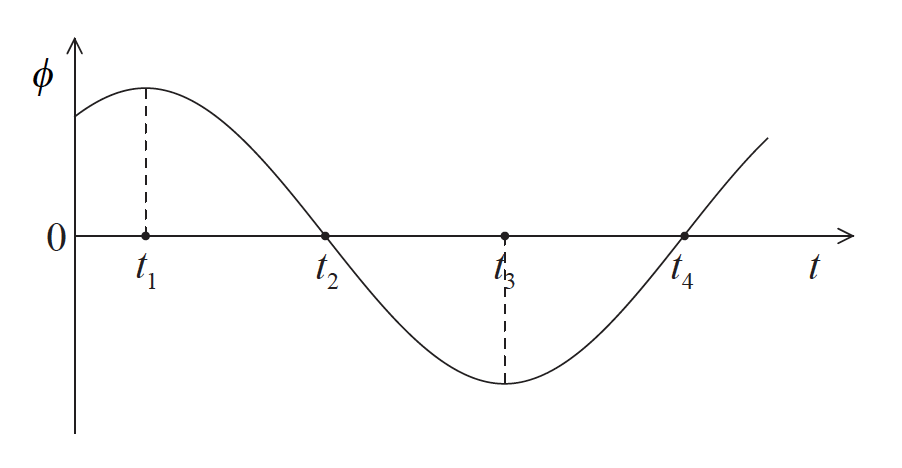The magnitude of the emf induced across the ends of the coil is maximum at time(s)

44. The peak value of an alternating sinusoidal potential difference is 100V. The approximate rms value of the potential difference will be
45. A parallel plate capacitor is connected to a cell of negligible internal resistance.The energy stored in the capacitor is 4 J and the electric field in between the plates is 100 N C–1. The distance between the plates of the capacitor is doubled. What are the energy stored and the electric field strength?